# Solving Multi-Step Inequalities

Multi-step inequalities are solved just like multi-step equations except that you have to be aware of when to reverse the inequality symbol. The symbol should be reversed if:

1)You reverse the sides of the equation
or
2)You multiply or divide both sides by a negative number.

Examples:

1) 3x - 4 < 5

3x - 4 + 4 < 5 + 4

3x < 9x < 3The symbol is never switched.

2) 2(x - 3) - 4x > 8

2x - 6 - 4x > 8

-2x - 6 > 8

-2x - 6 + 6 > 8 + 6 Notice the symbol does not switch here because we are adding.

-2x > 14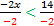The symbol switches because we are dividing by a negative number.

x < -7

3)2y - 8≤6y + 4

2y-6y- 8 ≤ 6y - 6y + 4

2y - 6y - 8≤ 4

-4y - 8≤ 4

-4y - 8 + 8≤ 4 + 8

-4y ≤ 12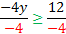y ≥ - 3 Notice the symbol only switched when we divided by a negative number

Practice: Solve the following inequalities.

1) -5x + 1 ≤ 4

2) 3y + 6y + 1 > - 8

3) 2(x - 4) + 3x < 6

4) 3y + 1 ≥ 8y - 4

5) - 3x + 5x - 2 > - 4x + 1

Answers:1)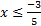2) y > - 13)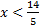4) y ≤ 15)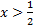Related Links: Math Algebra Inequalities Algebra Topics Graphing linear inequalities Solving inequalities with addition Solving inequalities with multiplication/division Solving multi-step inequalities Solving Inequalities